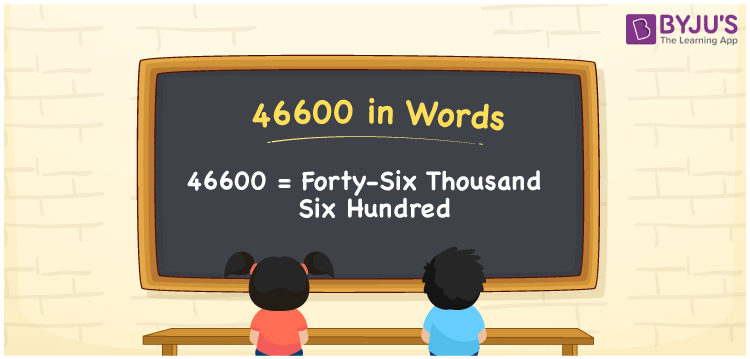# 46600 in Words

46600 in words is written as Forty-six thousand six hundred. In both the International System of Numerals and the Indian System of Numerals, 46600 is written as Forty-six thousand six hundred. The number 46600 is a Cardinal Number as it represents some quantity. For example, “that laptop costs 46600 rupees”.

 46600 in Words Forty-six thousand six hundred Forty-six thousand six hundred in Number 46600

## 46600 in English Words

46600 in English words is read as “Forty-six thousand six hundred.”## How to Write 46600 in Words?

To write 46600 in words, we shall use the place value chart. In the place value chart, put 4 in the ten thousands, 6 in the thousands, 6 in the hundreds and put 0 in the rest. Let us make a place value chart to write the number 46600 in words.

 Ten Thousands Thousands Hundreds Tens Ones 4 6 6 0 0

Thus, we can write the expanded form as

4 × Ten Thousand + 6 × Thousand + 6 × Hundred + 0 × Ten + 0 × One

= 4 × 10000 + 6 × 1000 + 6 × 100 + 0 × 10 + 0 × 1

= 40000 + 6000 + 600 + 0 + 0

= 46600

= Forty-six thousand six hundred.

46600 is a natural number, the successor of 46599 and the predecessor of 46601.

46600 in words – Forty-six thousand six hundred

• Is 46600 an odd number? – No
• Is 46600 an even number? – Yes
• Is 46600 a perfect square number? – No
• Is 46600 a perfect cube number? – No
• Is 46600 a prime number? – No
• Is 46600 a composite number? – Yes

## Frequently Asked Questions on 46600 in Words

Q1

### How to write 46600 in words?

46600 in words is written as Forty-six thousand six hundred.
Q2

### How to write 46600 in the International and Indian System of Numerals?

In both, the system of numerals, 46600 in words, is written as Forty-six thousand six hundred.
Q3

### How to write 46600 in a place value chart?

In the place value chart, write 4 in the ten thousands, 6 in the thousands, 6 in the hundreds and 0 in the tens and the ones, respectively.